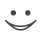# This flashcard is just one of a free flashcard set. See all flashcards!

174
14.Which of the following is true when dividends are expected?
A.Put-call parity does not hold
B.The basic put-call parity formula can be adjusted by subtracting the present value of expected dividends from the stock price
C.The basic put-call parity formula can be adjusted by adding the present value of expected dividends to the stock price
D.The basic put-call parity formula can be adjusted by subtracting the dividend yield from the interest rate